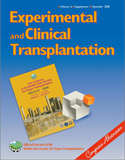## FULL TEXT

EXERCISE CAPACITY BEFORE LUNG TRANSPLANTATION: CORRELATES AND PREDICTORS

Exercise capacity, measured as the 6-minute walk test (6-MWT) result, is a determining factor in lung transplantation based on which a patients is judged to be transplanted or not. We investigated to see which factors are correlated to and which are predictors of exercise capacity in lung transplantation candidates. Data regarding 6-MWT along with demographic data, spirometry test results and some biochemical laboratory findings were extracted from lung transplantation waiting list database at Masih Daneshvari Hospital, Tehran, Iran. We used independent sample t-test and one-way ANOVA to compare findings between two or more groups of data. We used Pearson correlation coefficient to find which of the variables are significantly correlated with 6-MWT and used a linear regression model to see which of them are the predictor of 6-MWT. Data of the 88 patients has been extracted. 62(71%) of the patients were female. the mean age of the patients was 38.4 ± 16.1 years. the cause of the disease was bronchiectasia (39.8%), COPD (26.1%), pulmonary fibrosis (17%), primary pulmonary hypertension (12.5%) and other causes (4.5%). The mean 6-MWT was 271.7 ± 124.6 meters. There were no significant differences in exercise capacity among groups based on gender or disease. Age (R=-0.282, p=0.008), basal O2 saturation (R=0.322, p=0.002), FEV1 (R=0.217, p=0.046), FVC (R=0.239, p=0.028), PI-MAX(R=0.379, p=0.013) and BMI(R=-0.215, p=0.047) were all significantly correlated with 6-MWT. After entering the regression model, basal O2 saturation (B= 3.58, p<0.001), age (B= -2.37, p= 0.002) and FVC (B= 1.58, p= 0.033) were the predictors of 6-MWT (R2= 0.865, p < 0.001). In conclusion, we found that higher basal O2 saturation, younger age and higher FVC are predictors of better exercise capacity which could lead to better outcome after transplantation.Volume : 6
Issue : 4
Pages : 194

Transplant Research Center, Massih Daneshvari Hospital, Darabad-Niavaran, Tehran, Iran